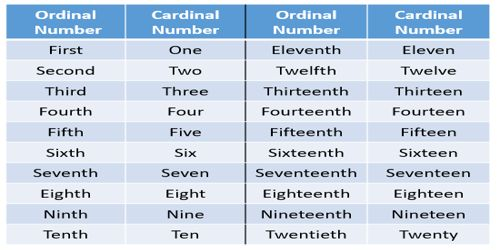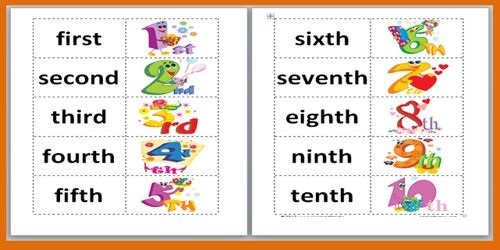Mathematic

# Ordinal NumberAn Ordinal Number is a natural number used for ordering (e.g. 1st, 2nd, 3rd, …). It is a number that tells the position of something in a list, such as 1st, 2nd, 3rd, 4th, 5th etc.

In formal set theory, an ordinal number (sometimes simply called an “ordinal” for short) is one of the numbers in Georg Cantor’s extension of the whole numbers. An ordinal number is defined as the order type of a well ordered set. It is easy to see that every finite totally ordered set is well ordered. Any two totally ordered sets with k elements (for k a nonnegative integer) are order isomorphic, and therefore have the same order type (which is also an ordinal number). The ordinals for finite sets are denoted 0, 1, 2, 3, …, i.e., the integers one less than the corresponding nonnegative integers.An ordinal number is used to describe the order type of a well ordered set (though this does not work for a well ordered proper class). A well ordered set is a set with a relation > such that

For any elements x and y, exactly one of these statements is true

• x > y
• y = x
• y > x

For any elements x, y, z, if x > y and y > z, x > z

Every nonempty subset has a least element, that is, it has an element x such that there is no other element y in the subset where x > y

Two well ordered sets have the same order type if and only if there is a bijection from one set to the other that converts the relation in the first set to the relation in the second set.

Explanation

Ordinal numbers do not indicate quantity as cardinal numbers do. Ordinal numbers attribute to a position or place of an object’s standing. They are written as first, second, third, or in numerals, as 1st, 2nd, and 3rd, etc. Normally, they are indicated by “th,” or sometimes by “nd” or “st.”

Look at these and notice the last two letters of the words

• 1st = first
• 2nd = second
• 3rd = third
• 4th = fourth
• 5th =fifth

Can you see the last two letters of the words make up the ordinal number?

• first – last two letters are ‘st’ so put after 1 = 1st
• second – last two letters are ‘nd’ put after 2 = 2nd
• third – last two letters are ‘rd’ so put after 3 = 3rd
• 4th – last two letters are ‘th’ so put after 4 = 4th

We just add ‘th’ to teen numbers:

• 14th = fourteenth (fourteen + th)
• 15th = fifteenth (It’s her fifteenth birthday.)
• 16th = sixteenth
• 17th = seventeenth
• 18th = eighteenth
• 19th = nineteenth.

In long numbers, ‘th’ is added to the last number:

• 106th = one hundred and sixth
• 242nd = two hundred and forty-secondth
• 2018th = two thousand and eighteenth.

Common Use of Ordinal Numbers

• He got third prize, sixth in line on his twentieth
• She was hired on January 8, and on the 25th she got appointment letter from board of directors.
• The first three series were wonderful to watch, but the second two were quite boring.
• Can’t you wait till March twenty-fourth?
• A boy of 20-years old, sitting with his twin brother remembering their eleventh birthday, which was celebrated with their family at an amusement park.